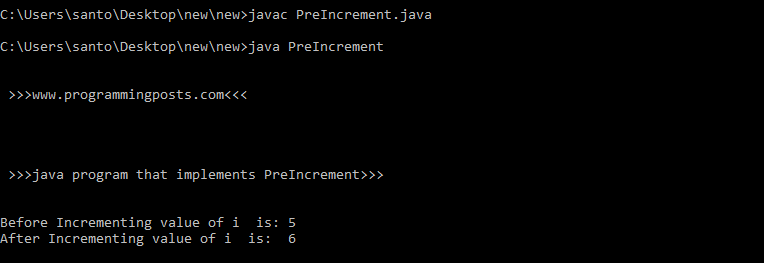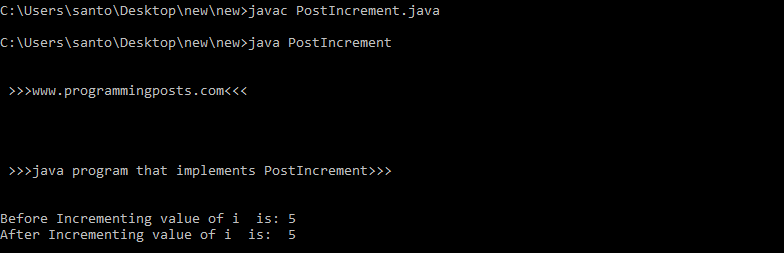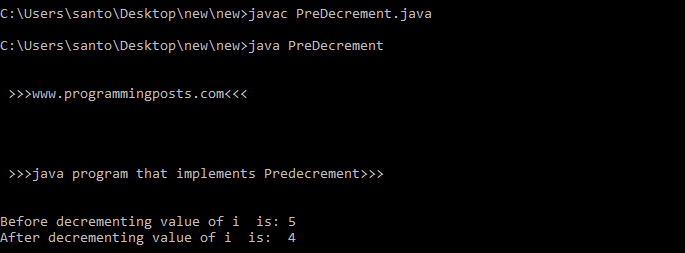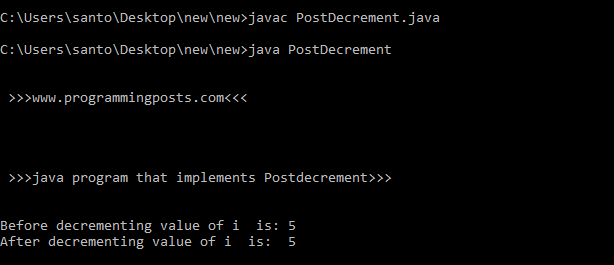# Java program for increment and decrement (pre and post)

Learning the operators of the Java programming language is a good place to start. Operators are special symbols that perform specific operations on one, two, or three operands, and then return a result.

### Increment/Decrement operators:

Increment and decrement operators are known as Unary operators. They require only one operand.

#### Increment operator:

Increment Operator is Used to Increment Value Stored inside Variable on which it is operating. it is similar to adding one to the variable. The Increment Operator is “++”.

#### Types of Increment Operator:

There are two types of Increment Operators.

1. preIncrement
2. postIncrement

#### preIncrement:

• “++” is written before the variable.
• Value is Incremented First and then incremented value is used in expression.
• “++” cannot be used over “constant” or “final variable”.

For example let us consider a variable i =3 . if the variable is preincremented using “++”  before the variable, the variable is incremented first and then it is assigned to the variable. The value becomes 4.

Lets see an example implementing PreIncrement Operator.

#### Source code:

```class PreIncrement
{
public static void main(String args[])
{
System.out.println("\n\n >>>www.programmingposts.com<<< \n\n");
System.out.println("\n\n >>>java program that implements PreIncrement>>> \n\n");
int i=5;
System.out.println("Before Incrementing value of i  is: "  +i);
int j = ++i;
System.out.println("After Incrementing value of i  is:  " +j);
}
}```

#### Explanation:

PreIncrement is the class which consists of main method in it.  An variable i of integer data type is taken and is assigned to 5. The variable i  is incremented using “++”  before i and is stored in j. Here the value is Incremented first by 1 and then the value is used as the expression.

#### Output:

C:\Users\santo\Desktop\new\new>javac PreIncrement.javaC:\Users\santo\Desktop\new\new>java PreIncrement

>>>www.programmingposts.com<<<

>>>java program that implements PreIncrement>>>

Before Incrementing value of i is: 5
After Incrementing value of i is: 6

#### Screenshot of  Output:Screenshot of Output

#### PostIncrement:

• “++” is written after the variable.
• Value is  First used in expression and then it is incremented.
• “++” cannot be used over “constant” or “final variable”.

For example let us consider a variable i =3 . if the variable is postincremented using “++”  after the variable, the value of the variable is used in the expression  first and then it’s value is incremented by 1.

lets see an example that implements PostIncrement Operator.

#### Source code:

```class PostIncrement
{
public static void main(String args[])
{
System.out.println("\n\n >>>www.programmingposts.com<<< \n\n");
System.out.println("\n\n >>>java program that implements PostIncrement>>> \n\n");
int i=5;
System.out.println("Before Incrementing value of i  is: "  +i);
System.out.println("After Incrementing value of i  is:  " + i++);
}
}```

#### Explanation:

PostIncrement is the class which consists of main method in it.  An variable i of integer data type is taken and is assigned to 5. The variable values is used first in the expression and is then incremented. The value is displayed on the screen.

In the above program the variable i is assigned to value 5. when it is post incremented, the value 5 is used in the expression that is the value 5 is displayed and then the value is incremented. after printing the  value it have the value 6.

#### output:

C:\Users\santo\Desktop\new\new>javac PostIncrement.javaC:\Users\santo\Desktop\new\new>java PostIncrement

>>>www.programmingposts.com<<<

>>>java program that implements PostIncrement>>>

Before Incrementing value of i is: 5
After Incrementing value of i is: 5

#### Screenshot of output:screenshot of output

#### Decrement operator:

Decrement Operator is Used to Decrement Value Stored inside Variable on which it is operating. it is similar to subtract one from the variable. The Decrement Operator is “–“.

#### Types of Decrement Operator:

There are two types of Decrement operator:

1. predecrement
2. postdecrement

#### predecrement operator:

• “–” is written before the variable.
• Value is decremented First and then decremented value is used in expression.
• “–” cannot be used over “constant” or “final variable”.

For example let us consider a variable i =3 . if the variable is predecremented using “–”  before the variable, the variable is decremented first and then it is assigned to the variable. The value becomes 2.

lets see an example program that implements predecrement operator.

### Source code:

```class PreDecrement
{
public static void main(String args[])
{
System.out.println("\n\n >>>www.programmingposts.com<<< \n\n");
System.out.println("\n\n >>>java program that implements Predecrement>>> \n\n");
int i=5;
System.out.println("Before Incrementing value of i  is: "  +i);
System.out.println("After Incrementing value of i  is:  " + --i);
}
}```

#### Explanation:

Predecrement is the class which consists of main method in it.  An variable i of integer data type is taken and is assigned to 5. The variable i  is decremented using “–”  before the variable i. Here the value is decremented first by 1 and then the value is used as the expression.

#### Output:

C:\Users\santo\Desktop\new\new>javac PreDecrement.javaC:\Users\santo\Desktop\new\new>java PreDecrement

>>>www.programmingposts.com<<<

>>>java program that implements Predecrement>>>

Before decrementing value of i is: 5
After decrementing value of i is: 4

#### Screenshot of Output:Screenshot of Output

#### PostDecrement Operator:

• “–” is written after the variable.
• Value is  First value is used in expression and then decremented its value by 1.
• “–” cannot be used over “constant” or “final variable”.

For example let us consider a variable i =3 . if the variable is postdecremented using “–”  after the variable, the value of the variable is used in the expression  first and then it’s value is decremented by 1.

lets see an example that implements Postdecrement operator.

```class PostDecrement
{
public static void main(String args[])
{
System.out.println("\n\n >>>www.programmingposts.com<<< \n\n");
System.out.println("\n\n >>>java program that implements Postdecrement>>> \n\n");
int i=5;
System.out.println("Before decrementing value of i  is: "  +i);
System.out.println("After decrementing value of i  is:  " + i--);
}
}```

#### Explanation:

PostDecrement is the class which consists of main method in it.  An variable i of integer data type is taken and is assigned to 5. The variable values is used first in the expression and is then Decremented. The value is displayed on the screen.

In the above program the variable i is assigned to value 5. when it is post decremented, the value 5 is used in the expression that is the value 5 is displayed and then the value is decremented. after printing the  value it have the value 6.

#### Output:

C:\Users\santo\Desktop\new\new>javac PostDecrement.javaC:\Users\santo\Desktop\new\new>java PostDecrement

>>>www.programmingposts.com<<<

>>>java program that implements Postdecrement>>>

Before decrementing value of i is: 5
After decrementing value of i is: 5

#### Screenshot of Output:Screenshot of output